##### Actions

A type of arrangement of the trajectories in a neighbourhood of a singular pointof an autonomous system of second-order ordinary differential equations(*), whereis the domain of uniqueness. This type is characterized as follows. Suppose that a certain neighbourhoodofis partitioned into(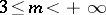) curvilinear sectors (cf. Sector in the theory of ordinary differential equations) by semi-trajectories (the separatrices of the saddle node) approaching. Suppose thatof these sectors,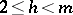, are saddle sectors and that the others are open nodal sectors, and suppose also that each semi-trajectory approaching, completed with, touches it in a definite direction. Thenis called a saddle node.

A saddle node is unstable in the sense of Lyapunov (cf. Lyapunov stability). Its Poincaré index is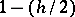(cf. Singular point). If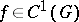and the matrix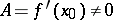, then the singular pointcan be a saddle node for (*) only when the eigenvalues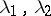ofsatisfy one of the following conditions:

a)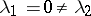;

b)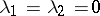.

In any of these casescan also be a saddle or a node for (*), and in case b), also a point of another type. If it is a saddle node, then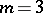,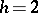, and all the semi-trajectories of the system that approachtouch at this point the directions defined by the eigenvectors of(see Fig. aand Fig. b, where the heavy lines are the separatrices at the saddle node, and the arrows indicate the direction of motion along the trajectories of the system asincreases; they can also be in the opposite direction).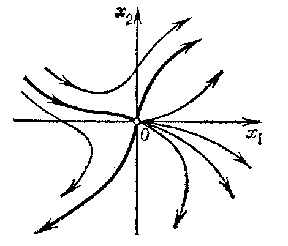Figure: s083040a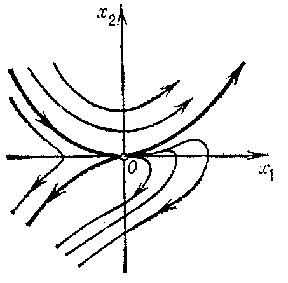Figure: s083040b